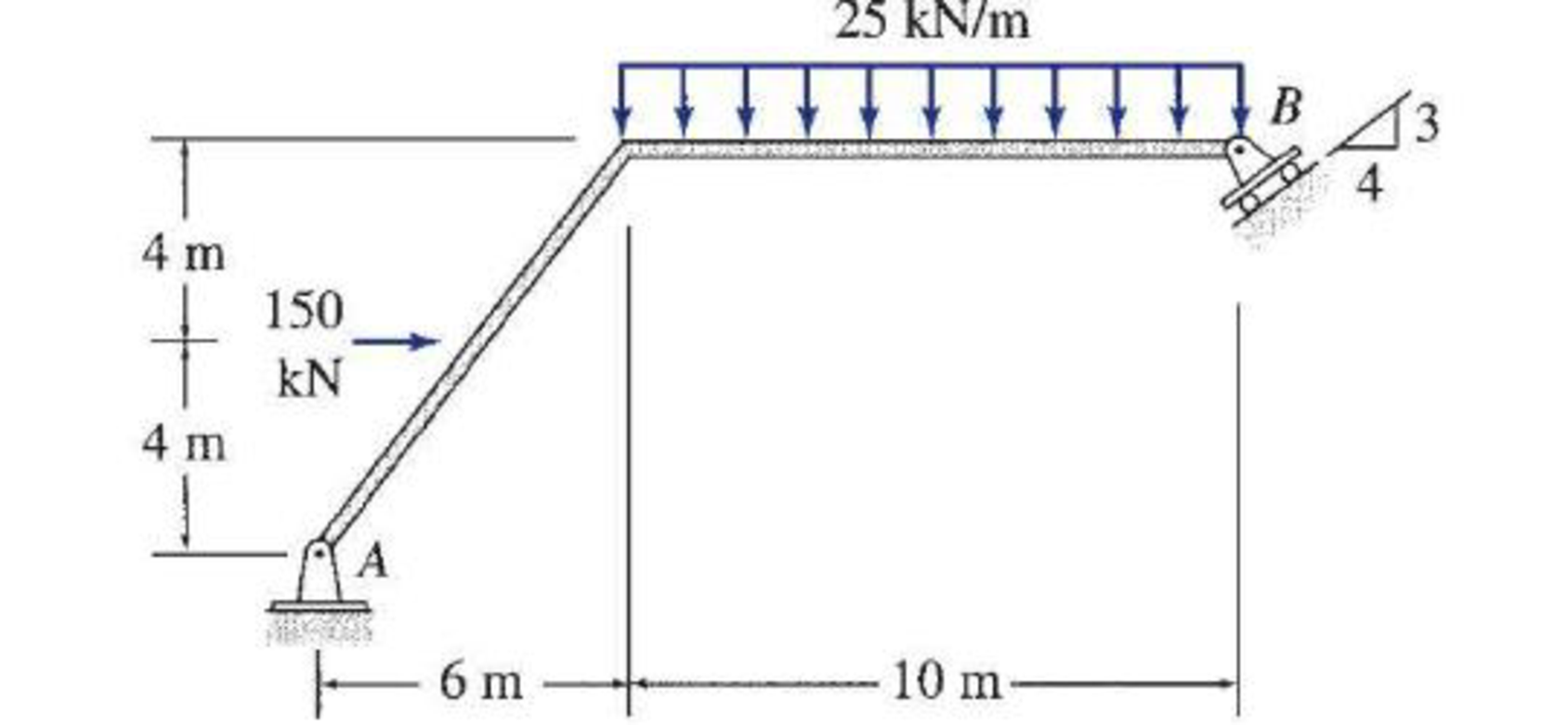# Determine the reactions at the supports for the structures shown. FIG.P3.24

#### Solutions

Chapter
Section
Chapter 3, Problem 24P
Textbook Problem
4 views

## Determine the reactions at the supports for the structures shown.FIG.P3.24

To determine

Calculate the support reactions for the given beam.

### Explanation of Solution

Given information:

The structure is given in the Figure.

Apply the sign conventions for calculating reaction forces and moments using the three equations of equilibrium as shown below.

• For summation of forces along x-direction is equal to zero (Fx=0), consider the forces acting towards right side as positive (+) and the forces acting towards left side as negative ().
• For summation of forces along y-direction is equal to zero (Fy=0), consider the upward force as positive (+) and the downward force as negative ().
• For summation of moment about a point is equal to zero (Matapoint=0), consider the clockwise moment as negative and the counter clockwise moment as positive.

Calculation:

Let the roller at B exerts the reaction RB in the direction perpendicular to the inclined supporting surface.

Let Ax and Ay be the horizontal and vertical reactions at the hinged support A.

Sketch the free body diagram of the beam as shown in Figure 1.

Use equilibrium equations:

Summation of moments about A is equal to 0.

MA=0150(4)25(10)(102+6)+35RB(8)+45RB(16)=03,350+4.8RB+12.8RB=017.6RB=3,350

RB=190

### Still sussing out bartleby?

Check out a sample textbook solution.

See a sample solution

#### The Solution to Your Study Problems

Bartleby provides explanations to thousands of textbook problems written by our experts, many with advanced degrees!

Get Started

Find more solutions based on key concepts
Name the different layers that make up the Earth.

Engineering Fundamentals: An Introduction to Engineering (MindTap Course List)

What are three safety rules to observe when using screwdrivers?

Precision Machining Technology (MindTap Course List)

How has computer security evolved into modern information security?

Principles of Information Security (MindTap Course List)

What are the three types of results that a subquery can return?

Database Systems: Design, Implementation, & Management

Identify the role of a bus. Differentiate between a data bus and an address bus.

Enhanced Discovering Computers 2017 (Shelly Cashman Series) (MindTap Course List)

How does the role of main memory differ from the role of secondary storage?

Principles of Information Systems (MindTap Course List)

Give one advantage of using electrodes with cellulose-based fluxes.

Welding: Principles and Applications (MindTap Course List)

If your motherboard supports ECC DDR3 memory, can you substitute non-ECC DDR3 memory?

A+ Guide to Hardware (Standalone Book) (MindTap Course List)